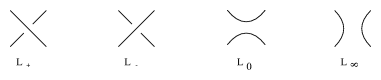# Brandt-Lickorish-Millett-Ho polynomial

An invariant of non-oriented links in $\mathbf{R}^3$, invented at the beginning of 1985 [a1], [a2] and generalized by L.H. Kauffman (the Kauffman polynomial; cf. also Link).
It satisfies the four term skein relation for a Kauffman skein quadruple (cf. also Conway skein triple) $$Q_{L_{+}}(z) + Q_{L_{-}}(z) = z\left({ Q_{L_{0}}(z) + Q_{L_{\infty}}(z) }\right)$$ and is normalized to be $1$ for the trivial knot.